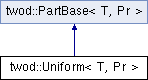# twod::Uniform< T, Pr > Class Template Reference

Implements the area based rectilinear of the space. More...

`#include <twod/uniform_2d.hpp>`

Inheritance diagram for twod::Uniform< T, Pr >:## Detailed Description

### template<typename T, typename Pr> class twod::Uniform< T, Pr >

Implements the area based rectilinear of the space.

The number of processors in each dimension can be controlled by setP().

Parameters:
 T type of element of the array Pr type of the prefix sum array

List of all members.

## Public Member Functions

Uniform ()
number of part in the first dimension. if 0, automatic
void setP (int P)
selects the number of stripes in the first dimension
virtual T part (int procCount, const Pr &prefixSumArray, util::RectList< T, Pr > &parts)
Applies a 2d partitioning algorithm to a given 2d prefixSumArray .
virtual ~Uniform ()

int P

## Constructor & Destructor Documentation

template<typename T , typename Pr >
 twod::Uniform< T, Pr >::Uniform ( ) ` [inline]`

number of part in the first dimension. if 0, automatic

template<typename T , typename Pr >
 virtual twod::Uniform< T, Pr >::~Uniform ( ) ` [inline, virtual]`

## Member Function Documentation

template<typename T , typename Pr >
 T twod::Uniform< T, Pr >::part ( int procCount, const Pr & prefixSumArray, util::RectList< T, Pr > & parts ) ` [virtual]`

Applies a 2d partitioning algorithm to a given 2d prefixSumArray .

Parameters:
 [in] procCount is the number of processors [in] prefixSumArray first column and first row consists of zero only. But the borders of rectangles in rect_list never touch this area (index 0 in row or column) [out] parts must be empty before calling this function. It will contain the partition after the function return
Returns:

Implements twod::PartBase< T, Pr >.

template<typename T , typename Pr >
 void twod::Uniform< T, Pr >::setP ( int P ) ` [inline]`

selects the number of stripes in the first dimension

Parameters:
 P number of stripes in the first dimension. If 0, the number of stripes will be computed at runtime by taking the square root of the number of processors.

## Member Data Documentation

template<typename T , typename Pr >
 int twod::Uniform< T, Pr >::P` [private]`

The documentation for this class was generated from the following files: